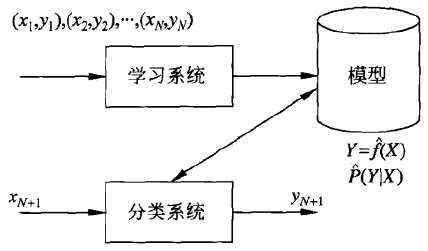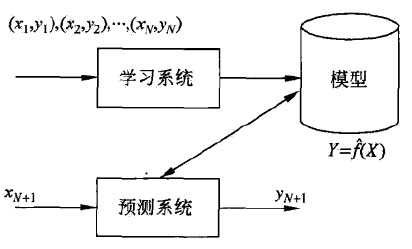# 概述

## 1. 定义

• 生成方法由数据学习联合概率分布 $P(X, Y)$，然后求出条件概率分布 $P(Y | X)$ 作为预测的模型，即生成模型：

$P(Y | X) = \frac{P(X, Y)}{P(X)}$

生成模型表示了给定输入 $X$ 产生输出 $Y$ 的生成关系。典型的生成模型有朴素贝叶斯法和隐马尔可夫模型。

• 判别方法由数据直接学习决策函数 $f(X)$ 或者条件概率分布 $P(Y | X)$ 作为预测的模型，即判别模型。判别方法关心的是对给定的输入 $X$，应该预测什么样的输出 $Y$典型的判别模型有：$k$ 近邻法、感知机、决策树、逻辑斯谛回归模型、最大熵模型、支持向量机、提升方法和条件随机场等。

## 2. 分类问题

• 在学习过程中，根据已知的训练数据集利用有效的学习方法学习一个分类器；
• 在分类过程中，利用学习的分类器对新的输入实例进行分类。• $TP$：将正类预测为正类数；
• $FN$：将正类预测为负类数；
• $FP$：将负类预测为正类数；
• $TN$：将负类预测为负类数。

$P = \frac{TP}{TP + FP}$

$R = \frac{TP}{TP + FN}$

$\frac{2}{F_1} = \frac{1}{P} + \frac{1}{R} \\ F_1 = \frac{2TP}{2TP + FP + FN}$

## 3. 标注问题

• 训练数据集 $T=\left\{\left(x_{1}, y_{1}\right),\left(x_{2}, y_{2}\right), \cdots,\left(x_{N}, y_{N}\right)\right\}$，其中$x_{i}=(x_{i}^{(1)}, x_{i}^{(2)}, \cdots, x_{i}^{(n)})^{\top}, i=1,2, \cdots, N$ 是输入序列，$y_{i}=(y_{i}^{(1)}, y_{i}^{(2)}, \cdots, y_{i}^{(n)})^{\top}$是相应的输出序列，$n$ 是序列长度，一般 $n \ll N$

$P\left(Y^{(1)}, Y^{(2)}, \cdots, Y^{(n)} \mid X^{(1)}, X^{(2)}, \cdots, X^{(n)}\right)$## 4. 回归问题

• 训练数据集 $T=\left\{\left(x_{1}, y_{1}\right),\left(x_{2}, y_{2}\right), \cdots,\left(x_{N}, y_{N}\right)\right\}$，其中 $x_{i} \in \mathbf{R}^{n}$ 为输入，$y \in \mathbf{R}$ 是对应的输出，$i = 1, 2, \cdots, N$## 附录

• 《统计学习方法》by 李航